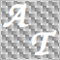• Übersicht
• Bewertungen (1)
• Diskussion (3)

Pairs Spread FreeVersion indicator is the free version of Pairs Spread Indicator offering the following instruments selections (EURUSD, EURGBP, AUDUSD, NZDUSD, AUDNZD) and can be also useful to traders testing the Pairs Trading EA by visualizing the error term of a tradable pair.

Pairs Spread indicator facilitates and makes easier the execution of a very popular speculative investment strategy used in Wall Street called pairs trading. In applying a pairs trading strategy, we are not interested in the price of each individual component but in the price difference between them. Pair’s Spread provides exactly this price difference or spread, making your trading decisions much easier (see Inputs section for more details). Moreover, Pairs Spread indicator has a Risk Management orientation with its two available Risk Management measures: Value at Risk (VaR) and Expected Shortfall (ES).

### Inputs

Let's assume that we have identified a long-term relationship between Microsoft and Intel corporation. Moreover, lets assume that after applying an econometric research, we have found that the long-term relationship between Microsoft and Intel is given by the following equation:

MSFT=-13.8362+2*INTC+error

Where the error is normally distributed with mean zero and unit standard deviation.

The above econometric equation uses D1 timeframe!

Given the above equation, the returned Pairs Spread price is:

P=MSFT-(-13.8362+2*INTC)

• Pair A symbol: Is the symbol of the first pair component. For example MSFT for the Microsoft Corporation. Its value should be a string.
• Pair B symbol: Is the symbol of the second pair component. For example INTC for the Intel Corporation. Its value should be a string.
• Mean: Is the long-term mean. In our example -13.8362. Its value is a double.
• Integrating Coefficient: Is the co-integrating coefficient. In our example 2. Its value is a double.
• First Threshold: It is the first threshold above and below the pair’s average and it is expressed in standard deviation terms, with its default value set to one standard deviation. Its value is a double. If its input value is greater than 5, then in runtime it will automatically be set to 1.
• Second Threshold: It is the second threshold above and below the pair’s average and it is also expressed in standard deviation terms, with its default value set to 1.25 standard deviations. Its value is a double. If its input value is greater than 5, then in runtime it will automatically be set to 1.25.
• Third Threshold: It is the third threshold above and below the pair’s average and it is also expressed in standard deviation terms, with its default value set to 2 standard deviations. Its value is a double. If its input value is greater than 5 then in runtime, it will automatically be set to 2.
• Number of Averaging Lags: Is the number of lags from which the average, thresholds, value at risk and expected shortfall will be derived. Its default value is set to 365. Its value should be an integer and should not exceed 600. If the input value is greater than 600, then in runtime it will be set to 365.
• Entry price: Is the entry pair’s spread price in either a long position or a short position. It will be used for estimating the Value at risk and the expected shortfall. A long position on a pair’s spread means buy pair a and sell pair b while a short position on a pair’s spread means sell pair a and buy pair b. In our example, the relation between the two pair components is ½, two stocks/lots of INTC for each MSFT stock/lot. In figure 1, the presented VaR and ES values for a SELL trade with entry price 2.0962 are 0.8425 and 1.5462 respectively. This means that for a short pair-position consisting of 1 lot short on MSFT and 2 lots long on INTC with contract size equal to 100 the total VaR and ES values are 0.8425*100=84.25 and 1.5462*100=154.62 respectively.
• Trade type: It could take either the BUY or SELL (in capital letters) value depending on our position on the pair’s spread. Its value should be a string.20961
2016.08.31 17:03

Der Benutzer hat keinen Kommentar hinterlassen